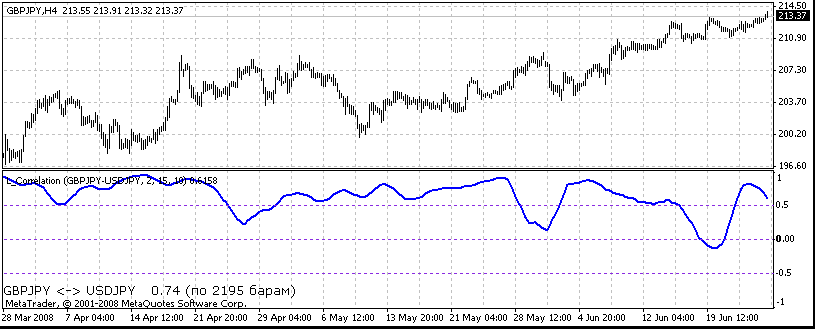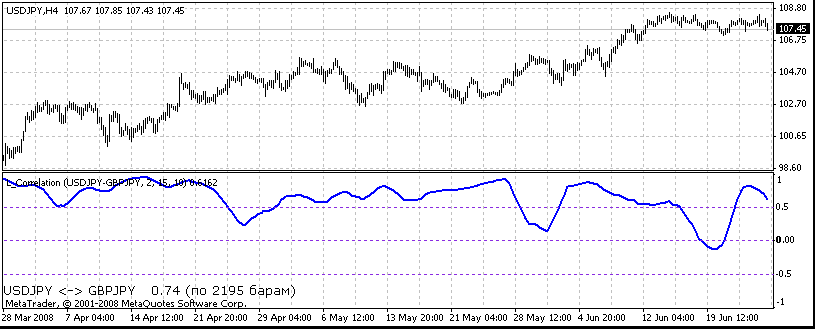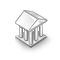Watch how to download trading robots for free
Interesting script?
So post a link to it -
let others appraise it
You liked the script? Try it in the MetaTrader 5 terminal# L_Correlation - indicator for MetaTrader 4

Views:
13999
Rating:
votes: 5
Published:
2008.06.28 17:12
Updated:
2014.04.21 14:52

The calculation of correlation coefficient between two quotes charts of two currency pairs is performed (between the currency pair to whose chart the indicator is attached and the pair specified in the parameters of the indicator).

The followings are displayed in the indicator's window:

• The chart of the correlation coefficient (you can disable its displaying as is shown on the mentioned pictures)

• The chart of the moving average related to the curve of the correlation coefficient (you can disable displaying)

• The average value of the correlation coefficient for the specified number of last barsMode = 0, 1, 2 - the parameter that determines the exact property of the price chart the correlation will be calculated for (0 - for the close price of the bar, 1- for the differnece between the open and the close prices, 2 - for the relation between the close price and the maximum price of the bar).

Pair = "USDCHF", "EURUSD"... - a currency pair the correlation coefficient is to be calculated for.
ShowCorrelation = true - if the displaying of the buffer of the correlation coefficient is necessary, = false - if not.
ShowMA = true - if the displaying of the buffer of the correlation coefficient's moving average is necessary, = false - if not.
CorrelationRadius = 15 - the radius of the correlation.
MA_Period = 10 - the period of the moving average.
ResultingBars = 0 - the number of bars the total average value of the correlation coefficient is calculated by (this value is displayed at the bottom-left part of the window). If ResultingBars = 0, then the average will be calculated by all obtained values of the correlation coefficient.

FontName = "Verdana" - the font the average value of the correlation coefficient is displayed with.
FontSize = 10 - the font size.
FontColor = Black - the font color.

Translated from Russian by MetaQuotes Software Corp.
Original code: https://www.mql5.com/ru/code/8224The pattern MACD - head and shoulders. Good but on rare occasions.

A trade strategy that functions with the pattern MACD "head and shoulders"ZigAndZag_trader

At Bookkeeper's request, I've made an "egzpert advizor" by the ZigAndZag indicator, published at: http://codebase.mql4.com/ru/3681Pendulum 1_01

The parameters of working - Places two starting pending orders for buying and selling (the size of the lot is calculated automatically on the basis of the deposit size and the maximum increase of leverage from 0.01 to 0.07 lots) taking into account thb-SharingDoW

The receiving of the EA testing results by the days of week.